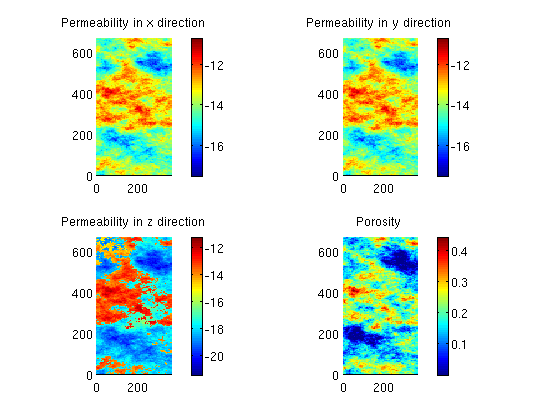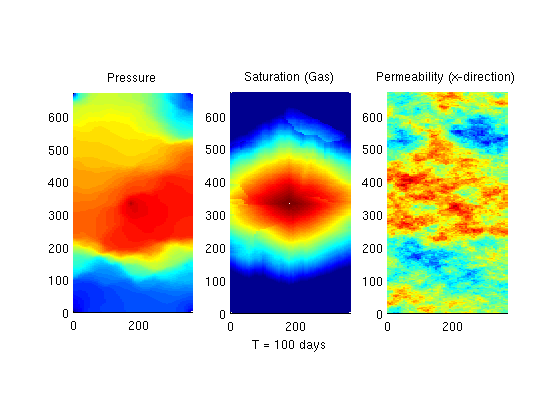#SPE10 Quarter Five Spot Example

## Document example dependcies

```require impes spe10 deckformat
```

## Define a oil/gas system

The fluid data is contained in the file 'simpleOilGas.txt'. We read and process this using the deckformat module.

```current_dir = fileparts(mfilename('fullpath'));
f = fullfile(current_dir, 'simpleOilGas.txt');

% Once the file has been read, we can use it to create the desired fluid.
fluid = initEclipseFluid(deck);
```

## Set up grid and get petrophysical properties.

We select three layers to

```layers = 25:28;
cartDims = [60, 220, numel(layers)];
physDims = cartDims.*[20,10,2]*ft;
rock = SPE10_rock(layers);
rock.perm = convertFrom(rock.perm, milli*darcy);

gravity off

% Define a Cartesian Grid based on the SPE layers selected.
G = cartGrid(cartDims, physDims);
G = computeGeometry(G);

% Cells with zero porosity are masked away to the minimum value to get
% sensible results.
is_pos = rock.poro>0;
rock.poro(~is_pos) = min(rock.poro(is_pos));
```

## Show grid and petrophysical properties

Since the variations are large, use a logarithmic scale for permeability

```perm = log10(rock.perm);
c = {'x', 'y', 'z'};
clf;
for i = 1:3
subplot(2,2,i);
plotCellData(G, perm(:,i));
colorbar();
axis equal tight;
title(sprintf('Permeability in %s direction', c{i}));
end
% Plot porosity
subplot(2,2,4);
plotCellData(G, rock.poro);
colorbar();
axis equal tight;
title('Porosity')
```## Set up four producers at reservoir pressure and an injector at 500 bar

```% Initialize empty well structure
W = [];

% Add an injector in the middle of the domain based on the midpoints of the
% cartesian indices
midpoint = round(cartDims)./2;

% Use vertical well, with completion in all layers. To complete in a
% different set of layers, enter the indices of the layers in completion
completion = [];
W = verticalWell(W, G, rock, midpoint(1), midpoint(2), completion, ...
'Type', 'bhp',...            % Bottom hole pressure well
'Val', 500*barsa,...         % Driving pressure
'Radius', .125*meter, ...    % As per SPE10 Case B
'InnerProduct', 'ip_tpf',... % Use TPFA inner product
'Comp_i', [0, 1]);           % Injects only gas

% We want to place an producer in each corner of the domain, so we iterate
% over the corners and add a 200 bar well at each corner.
h_ind = [1, G.cartDims(1)];
v_ind = [1, G.cartDims(2)];
for i = 1:2
for j = 1:2
W = verticalWell(W, G, rock, h_ind(i), v_ind(j), [], ...
'Type', 'bhp',...
'Val', 200*barsa, ...
'InnerProduct', 'ip_tpf', ...
'Comp_i', [1, 0]);
end
end
```

## Compute one time values for the IMPES solver

Compute TPFA transmissibilities

```Trans = computeTrans(G, rock);
% Compute pore volume
PV = poreVolume(G, rock);
```

## Initialize a oil filled reservoir at 200 bar.

Initially, it contains no gas

```x = initResSolComp(G, W, fluid, 200*barsa, [1, 0]);
```

## Do actual simulations

Simulate 100 days of production

```dT = 1*day;
Nt = 100;

f1 = figure(1);
T  = 0;
tic()
for kk = 1:Nt,
x = impesTPFA(x, G, Trans, fluid, dT, PV, 'wells', W);

T = T + dT;

% We skip drawing the values for the cells containing wells, since they
% are always outliers.
notPerf = ~ismember(1:G.cells.num, vertcat(W.cells));

set(0, 'CurrentFigure', f1);
clf;

% Plot the pressure.
subplot(1,3,1);
plotCellData(G, (x.pressure), notPerf);
title('Pressure')
axis equal tight;

% Plot the gas saturation
subplot(1,3,2);
plotCellData(G, log10(x.s(:,2)), notPerf);
title('Saturation (Gas)')
xlabel(sprintf('T = %1.0f days', convertTo(T, day)));
axis equal tight;

% Plot the x component of the permeability
subplot(1,3,3);
plotCellData(G, log10(rock.perm(:,1)));
title('Permeability (x-direction)')
axis equal tight;

drawnow

end
toc()
```
```Elapsed time is 168.679231 seconds.
```Published October 2, 2012